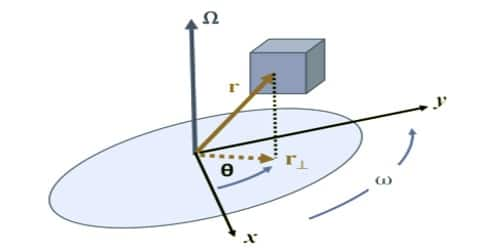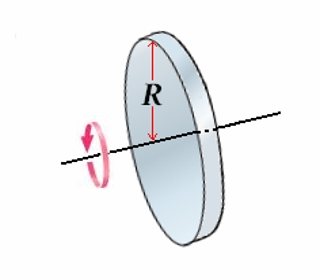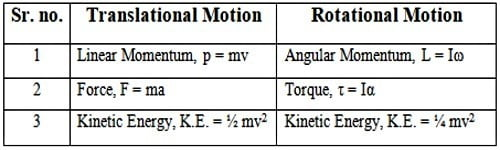Physics

# Moment of Inertia and its Physical SignificanceMoment of inertia is a determination of how complicated it is to rotate an exacting body about a given axis. It is a measure of how difficult it is to rotate a particular body about a given axis. According to Newton’s first law of motion, a body must continue in its state of rest or of uniform motion unless it is compelled by some external agency called force. The inability of a material body to change its state of rest or of uniform motion by itself is called inertia.

Inertia is the fundamental property of the matter. Moment of inertia is the mass property of a rigid body that determines, the torque needed for a desired angular acceleration about an axis of rotation. It is an inherent property of matter. It is due to the inertia, a body opposes any alteration in its state of rest or of uniform motion in a straight line. For a given force, the greater the mass, the higher will be the opposition to the motion or the larger the inertia. Thus, in translatory motion, the mass of the body measures the coefficient of inertia.Similarly, in rotational motion also, a body, which is free to rotate about a given axis, opposes any change desired to be produced in its state. The measure of opposition will depend on the mass of the body and the distribution of mass about the axis of rotation. The coefficient of inertia in rotational motion is called the moment of inertia of the body about the given axis.

Significance:

• Greater the mass concentrated away from the axis, superior the moment of inertia. Moment of inertia of a body is dissimilar with reverence to the different axis of rotation.
• It is considerable in rotatory motion. It is the property of the body due to which it opposes the change in state in rotatory motion.
• It is related to the rotational motion as mass is related to translational motion.

Physical Significance of Moment of Inertia:

The physical significance of the Moment of Inertia of a body about an axis is the allocation of the mass of the body in space about the axis. Greater the mass concentrated away from the axis, greater the moment of inertia. For example, take a baseball club. If you rotate it by holding the handle, you have to put in more effort than when you rotate it by holding it at the hitting end.

Moment of inertia plays the same role in rotational motion as that of mass in translatory motion. Thus the moment of inertia in the rotational motion is comparable to the mass in translational motion because it plays a similar function in rotational motion as the mass plays in translational motion. This is clear from the following table:Newton’s first law of motion is also called a law of inertia indicates that a body is unable to change by itself its state of rest or state of uniform motion along a straight line. This property of inertness is known as inertia. It is an inherent property of matter. It is due to the inertia, a body opposes any change in its state of rest or of uniform motion in a straight line.

It can also be described as the determination of the resistance of a body to an exterior torque, the similar method mass is the measure of the resistance of a body to an exterior force. Also, to bring about a change in the state of rotation, torque has to be applied. Thus the moment of inertia in the rotational motion is analogous to the mass in translational motion because it plays the same role in rotational motion as the mass plays in translational motion.